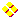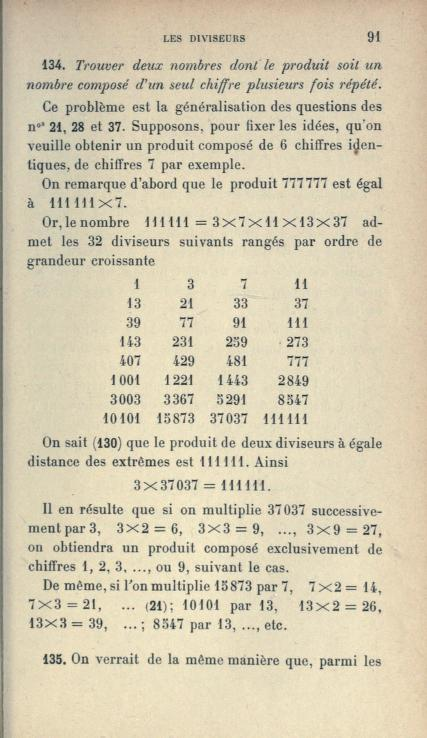World!Of
NumbersWON plate
110 |

[ July 16, 2001 ]
A Pandigital Programming Challenge based on sequence A061604
Amarnath Murthy (email)

A pandigital number is a number containing all ten digits from 0 to 9.
For the sake of this topic we allow  multiplicity  of the digits.

Amarnath Murthy submitted integer sequence A061604 to Sloane's
database in May 2001, displaying the smallest pandigital multiples of n.

multiplicand nmultiplier mpandigital n*m
110234567891023456789
25117283991023456798
33411522631023456789
42558644741023457896
52046935791023467895
61705761331023456798
71462081141023456798
81279322371023457896
91137174211023456789
101234567891234567890
11etc.etc.

As you can see all the pandigitals in the right column are ten (10) digits
long for these first few values of n. What I like to ask you now is to find
the smallest set (n,m) so that n*m is a pandigital number of at least
eleven (11) digits not ending in zero's.
I hope you enjoy this programming challenge !Nearly twenty one years later I decided to search for that smallest set
myself and wrote thereto the following Pari/gp program

```\\ Patrick De Geest [ GP/PARI CALCULATOR Version 2.11.4 ] February 11, 2022.
{
for(n = 1, oo,
m = 1023456789\n-1; fl=0;
while(fl==0,
m+=1; p=n*m;
p = vecsort(digits(p)); fl = 1;
for(i = 0, 9, fl *= vecsearch(p,i); );
if(fl, print(n," ",m," ",n*m," [",length(p),"]");
write("c:/pari/won110.txt", n," ",m," ",n*m," [",length(p),"]"));
);
);
}
```
It is a curious program as it starts very slow.
As n grows the speed accelerates ! So be a little patient at start.

Soon the program delivered our first minimal set (n=smallest , m).

37037 * 276094 = 10225693478
with an 11-digit pandigital!

100001 * 1235794 = 123580635794
with an 12-digit pandigital!

1000001 * 3245698 = 3245701245698
with an 13-digit pandigital!Observations

The multiplicands n for our 12-digit and 13-digit pandigitals
are 100001 & 1000001 are palindromic !Many of the multiplicands are multiples of 5291.
So is our n = 37037 which is 7 * 5291.
Inspect the entries in the OEIS sequence A178027.Patterns with 11-digit pandigitals (no multiples of 10)

Some n's are tautonymic !
148148 * 69093 = 10235989764
185185 * 55235 = 10228693475
259259 * 39442 = 10225693478
296296 * 34861 = 10329174856
370370 * 33345 = 12349987650
407407 * 25839 = 10526989473
etc.

Some n's are repdigital !
111111 * 92115 = 10234989765
222222 * 46062 = 10235989764
333333 * 30705 = 10234989765
444444 * 23031 = 10235989764
555555 * 18423 = 10234989765
666666 * 15354 = 10235989764
777777 * 13275 = 10324989675
888888 * 11673 = 10375989624
999999 * 10235 = 10234989765
etc.

Some n's are palindromic. Nice pattern!
110011 * 94069 = 10348624759
220022 * 49739 = 10943674258
330033 * 38166 = 12596039478
440044 * 28567 = 12570736948
550055 * 19975 = 10987348625
660066 * 19083 = 12596039478
770077 * 16324 = 12570736948
880088 * 14436 = 12704950368
990099 * 12722 = 12596039478

... palindromes outside the pattern
544445 * 20663 = 11249867035
649946 * 15931 = 10354289726
661166 * 15554 = 10283775964
713317 * 16927 = 12074316859
728827 * 14059 = 10246578793
797797 * 13257 = 10576394829
811118 * 12788 = 10372576984
859958 * 12487 = 10738295546
986689 * 10638 = 10496397582
988889 * 10469 = 10352678941
998899 * 12163 = 12149608537

Patterns with 12-digit pandigitals (no multiples of 10)

Some n's are palindromic. Nice pattern!
100001 * 1235794 = 123580635794
200002 * 617897 = 123580635794
300003 * 416231 = 124870548693
400004 * 309374 = 123750837496
500005 * 253679 = 126840768395
600006 * 209566 = 125740857396
700007 * 176542 = 123580635794
800008 * 154687 = 123750837496
900009 * 149521 = 134570245689

Here is another pattern!
1001001 * 122945 = 123068067945
1010101 * 103689 = 104736362589
1100011 * 116945 = 128640786395
1111111 * 92115 = 102349989765
but also
2002002 * 63469 = 127065064938
2020202 * 52098 = 105248483796
2200022 * 62036 = 136480564792
2222222 * 46062 = 102359989764
etc.

Some curious n's outside the pattern
1500015 * 88313 = 132470824695
1600016 * 89737 = 143580635792
1700017 * 127999 = 217600475983
1800018 * 107077 = 192740527386
1900019 * 67705 = 128640786395
2100021 * 59876 = 125740857396

Patterns with 13-digit pandigitals (no multiples of 10)

The beginning of a pattern ?
1000001 * 3245698 = 3245701245698
etc.

Some curious n's outside the pattern (but with 1 zero at the end)
1000010 * 1234685 = 1234697346850
2000020 * 617892 = 1235796357840
etc.This is page 91 of Emile Fourrey's book from 1899, Librairie Nony,
“Récréations arithmétiques”
where he expands on the numbers 5291 and 37037A000110Prime Curios!Prime PuzzleWikipedia 110Le nombre 110```

```

[TOP OF PAGE]

Patrick De Geest - Belgium- Short Bio - Some Pictures